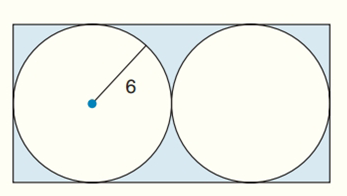Chapter 8.CR, Problem 30CR### Elementary Geometry for College St...

6th Edition
Daniel C. Alexander + 1 other
ISBN: 9781285195698

#### Solutions

Chapter
Section### Elementary Geometry for College St...

6th Edition
Daniel C. Alexander + 1 other
ISBN: 9781285195698
Textbook Problem
1 views

# Find the exact areas of the shaded regions in Exercises 27 to 31.Two ≅ tangent circles, inscribed in a rectangle.

To determine

To find:

The exact areas of the shaded regions.

Explanation

Calculation:

Consider the two tangent circles are shown below:

The above two tangent circles inscribed in a rectangle are congruent.

That is radius and areas of the two circles are same.

Area of the first circle A=πr2

A=π(6)2=36π

The total area of the two circles = Area of the first circle + Area of the second circle

=36π+36π=72π

To find the area of the rectangle:

The area A of a rectangle whose base has length b and whose altitude has length h is given by

### Still sussing out bartleby?

Check out a sample textbook solution.

See a sample solution

#### The Solution to Your Study Problems

Bartleby provides explanations to thousands of textbook problems written by our experts, many with advanced degrees!

Get Started

#### Find the domain of the function f(x)=142xx2.

Precalculus: Mathematics for Calculus (Standalone Book)

#### (exex2)1/2

Applied Calculus for the Managerial, Life, and Social Sciences: A Brief Approach

#### True or False: is a convergent series.

Study Guide for Stewart's Multivariable Calculus, 8th

#### Self Check Solve: x3+73=x+1.

College Algebra (MindTap Course List)